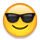# Running Total Line Chart using Beastmode CalculationContributorI am trying to use a line chart to display the running total of a beast mode calculation but I have not been able to find the correct solution. The beast mode calculation is a simple ratio, SUM(Column A)/SUM(Column.  The issue I am having is that the beast mode calculates separately for each period depending on the date grain used for the chart and then aggregates the calculated ratios for each period for the running total which is not what I need.  I need the ratio to be calculated each month based on the YTD data rather than each month independently.  Is this possible?